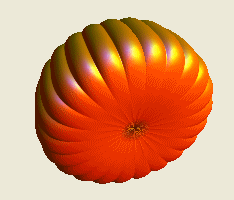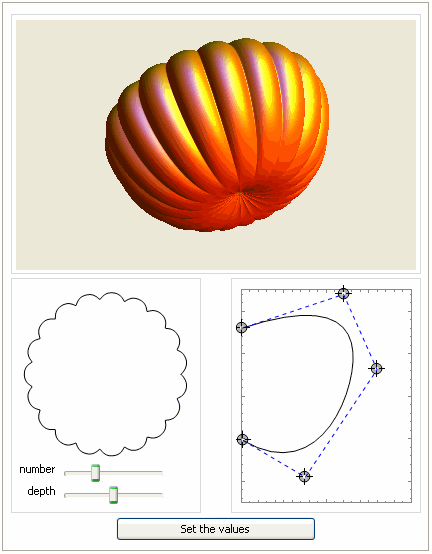Search IntMath
Close

450+ Math Lessons written by Math Professors and Teachers

5 Million+ Students Helped Each Year

1200+ Articles Written by Math Educators and Enthusiasts

Simplifying and Teaching Math for Over 23 Years

# Math-inspired Halloween Pumpkin

By Murray Bourne, 28 Oct 2012

In the spirit of Halloween, Yu-Sung Chang of Wolfram used Mathematica to "carve" a mathematically generated pumpkin.

Chang explains the process of creating the 3-D pumpkin shape using Mathematica. He shows how the overall chape (vertical cross-section) and indentations (seen in the horizontal cross-section) were developed via the use of B-splines. (A spline is a smooth curve passing through several points, and a B-spline is a generalization of the better-known Bezier Curve.)

The article includes a CDF (computable document format) download, so you can tweak certain parameters.

Here is the base of Chang's resulting pumpkin.I felt it was too flat, and in the above screen shot, it looks a bit like a space capsule re-entering the Earth's atmosphere!

So I tweaked the cross-sectional spline giving a pumpkin with a bit more natural shape. Here's the result.In the above screen shot from the CDF, you can see the cross-section with sliders that allow you to change the number and depth of the ribs of the pumpkin. At the lower right you can see the spline shape which is rotated about the contral axis to produce the pumpkin.

Chang then explains how to carve out a shape in our mathematically-generated pumpkin, using a texture-mapping technique.

Here's the result.Go check out the original Wolfram blog article and be sure to download the Computable Document Format (CDF) file so you can make your own adjustments to the pumpkin. (You'll need the CDF player plug-in which is certainly worth having because you can then play with many other math interactives on the Wolfram site.)

Happy Halloween!

### 2 Comments on “Math-inspired Halloween Pumpkin”

1. Alan Belniak says:

This is awesome! I work at PTC (full disclosure) and we had a similar contest last year. We challenged our users to model a spooky jack o' lantern using 3D CAD software.

Your math approach is great! I hope to see more like this!

-Alan Belniak / @abelniak
PTC

2. Murray says:

Thanks for sharing, Alan. That's a great competition idea!

### Comment Preview

HTML: You can use simple tags like <b>, <a href="...">, etc.

To enter math, you can can either:

1. Use simple calculator-like input in the following format (surround your math in backticks, or qq on tablet or phone):
a^2 = sqrt(b^2 + c^2)
(See more on ASCIIMath syntax); or
2. Use simple LaTeX in the following format. Surround your math with $$ and $$.
$$\int g dx = \sqrt{\frac{a}{b}}$$
(This is standard simple LaTeX.)

NOTE: You can mix both types of math entry in your comment.